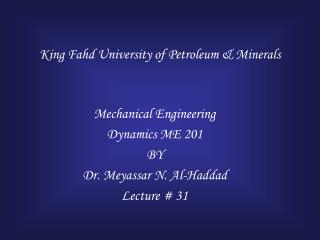# King Fahd University of Petroleum & Minerals - PowerPoint PPT PresentationDownload PresentationKing Fahd University of Petroleum & Minerals

King Fahd University of Petroleum & MineralsDownload Presentation## King Fahd University of Petroleum & Minerals

- - - - - - - - - - - - - - - - - - - - - - - - - - - E N D - - - - - - - - - - - - - - - - - - - - - - - - - - -
##### Presentation Transcript

1. King Fahd University of Petroleum & Minerals Mechanical Engineering Dynamics ME 201 BY Dr. Meyassar N. Al-Haddad Lecture # 31

2. Objectives • Apply the Equation of Translation motion • Rectilinear Translation • Curvilinear Translation • Apply the equation of Rotation motion • The roll of the center of mass G • Discuss the slipping Vs. Tipping • Discuss the slipping Vs. freely rotating • Discuss the “wheely” Vs. Non-wheely

3. General Application of the Equations of Motion Summation of moment in FBD = summation of the kinetic moment in K.D

4. Kinetic Moment

5. Rectilinear Translation

6. Curvilinear Translation

7. x N slipping Vs. Tipping If x > 1.5 ft tipping If x < 1.5 ft slipping

8. Slipping Vs. Freely rotating Front wheel Vs Back wheel drive Vs Four wheel drive mg FA=msNA NA NB slipping Freely Rotating

9. “Wheely” Vs. Non-Wheely Wheely : lift the front wheel off the ground NB=0

10. Example 17-5

11. Example mk=0.2 a= ? Flip Or Tipp? If x > 0.5 m tipping will take place

12. Example mm=125 kg mR= 75 kg a= ? NB=? FB=? ms=? Minimum to Lift front wheel

13. Problem aG mBNB NA NB m = 80 kg mB= 0.8 NA= ? NB= ? When rear wheel locks for break a = ? Deceleration

14. Problem a = 0 mBNB NA NB m= 80 kg mB= ? NA=? NB=? a =? When traveling at constant velocity and no break was applied

15. Problem mkNA NA NB=0 m= 80 kg NA=? NB=? a =? mk=? minimum When rider applies the front break and back wheel start to lift off the ground

16. Example mBD=100kg mAB=mCD= Neglect q = 30o w = 6 rad/s TA= ? TD= ? aG=?

17. Thank You